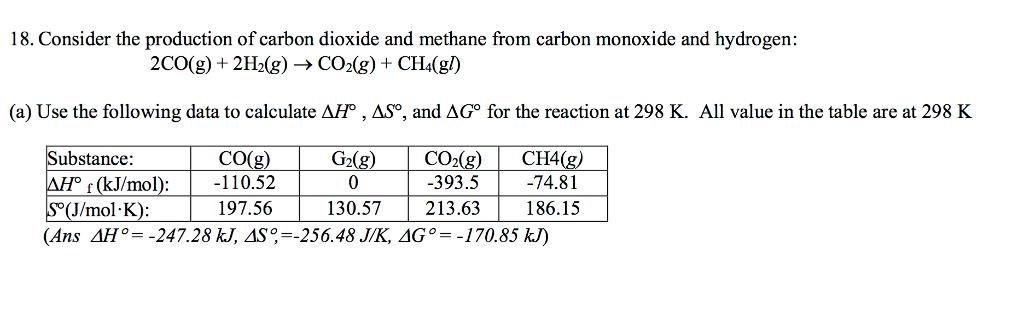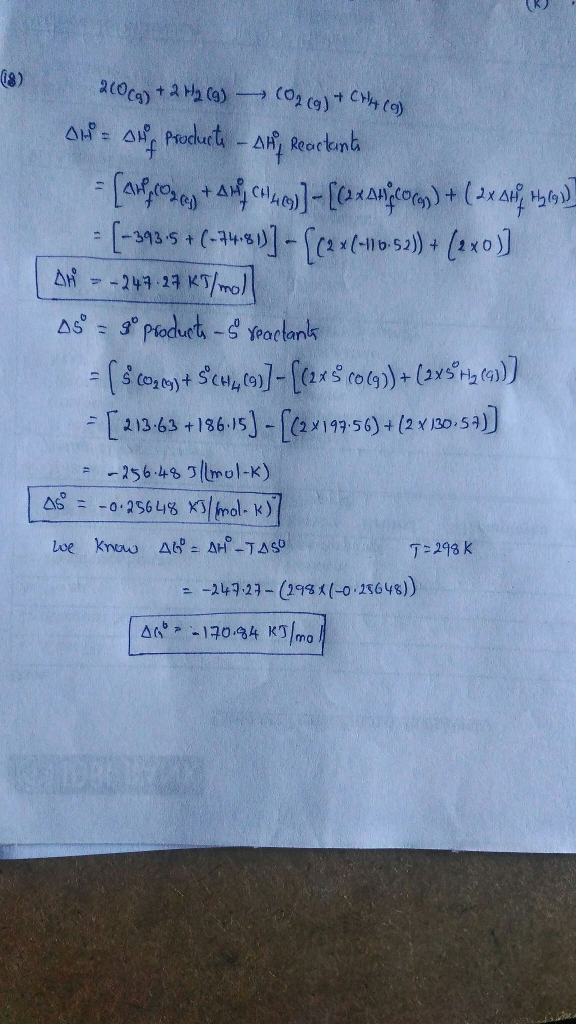# Question & Answer: Consider the production of carbon dioxide and methane from carbon monoxide and hydrogen…..Consider the production of carbon dioxide and methane from carbon monoxide and hydrogen: 2CO(g) + 2H_2(g) rightarrow CO_2(g) + CH_4(gl) (a) Use the following data to calculate Delta H degree, Delta S degree, and Delta G degree for the reaction at 298 K. All value in the table are at 298 K (Ans Delta H degree = -247.28 kJ, Delta S degree, = -256.48 J/K, Delta G degree = -170.85 kJ)